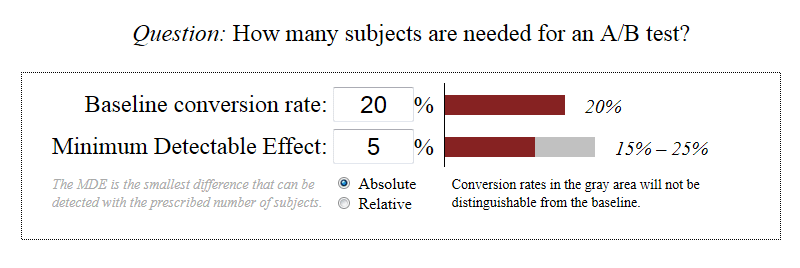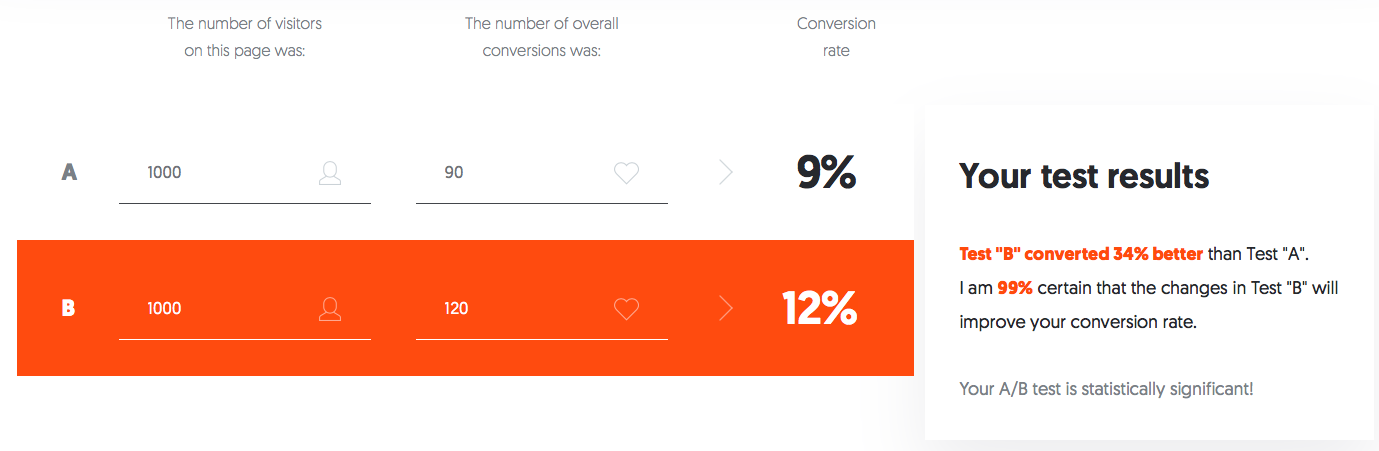# How A/B Testing Works (for Non-Mathematicians)

A/B testing is a great way to determine which variation of a marketing message will improve conversion rates (and therefore likely improve sales and revenue).

Many of you use A/B testing already, but you may need some help understanding what all the results mean. My goal here is to explain the numbers associated with A/B testing without getting bogged down in mathematical equations and technical explanations.

A/B testing results are usually given in fancy mathematical and statistical terms, but the meanings behind the numbers are actually quite simple. Understanding the core concepts is the important part. Let the calculators and software do the rest!

## Sampling and Statistical Significance

The first concept to discuss is sampling and sample size. Determining whether the results from a set of tests are useful is highly dependent on the number of tests performed. The measurement of conversion from each A/B test is a sample, and the act of collecting these measurements is called sampling.Let’s suppose you own a fast food restaurant and would like to know if people prefer French fries or onion rings. (If you are already in business, you probably know the answer from sales of each.) Let’s pretend you are not in business yet and want to estimate which will sell more, so you can pre-order your stock of each accordingly.

Now, suppose you conduct a survey of random people in the town where the restaurant will be located, and you ask them which they prefer. If you ask only three people total, and two say they like onion rings better, would you feel confident that two-thirds of all customers will prefer onion rings, and then order inventory proportionately? Probably not.

As you collect more measurements (or samples, and in this case, ask more people), statistically the results stabilize and get closer to representing the results you will actually see in practice. This applies just as much to website and marketing strategy changes as it does to French fries and onion rings.

The goal is to make sure you collect enough data points to confidently make predictions or changes based on the results. While the math behind determining the appropriate number of samples required for significance is a bit technical, there are many calculators and software applications available to help. For example, evanmiller.org has a free tool you can start using right now:## Confidence Intervals

It is likely that you have seen a confidence interval, which is a measure of the reliability of an estimate, typically written in the following form: 20.0% ± 2.0%.

Let’s suppose you performed the French fries-versus-onion-rings survey with an adequate number of people to ensure statistical significance, which you determined by using your trusty statistical calculator or software tool. (Note that the sample population (demographics, etc.) matters as well, but we will omit that discussion for simplicity.)

Let’s say the results indicated 20% of those surveyed preferred onion rings. Now, notice the ± 2.0% part of the confidence interval. This indicates the upper and lower bounds of the people who prefer onion rings and is called the margin of error. It is actually a measurement of the deviation from the true average over multiple repeated experiments.

Going back to the 2% margin of error, subtracting 2% from 20% gives us 18%. Adding 2% to 20% gives us 22%. Therefore, we can confidently conclude that between 18-22% of people prefer onion rings. The smaller the margin of error, the more confident we can be in our estimation of the average result.

Assuming a good sample population and size, this basically tells us we can confidently assume that if we were somehow able to survey, for example, everyone in the United States, 95% of the survey answers received in favor of onion rings would lie somewhere between 18-22%. In other words, we can be relatively certain that 18-22% of the people in the U.S. prefer onion rings over French fries.

Therefore, if we are placing an order to stock our restaurant, we may want to make sure that 22% of our onion rings-and-French-fries inventory is onion rings, and the rest is French fries (i.e., 78%). Then, it would be very unlikely we would run out of either, assuming the total stock is enough for the amount of time between orders.

## Confidence Intervals in A/B Testing

Applying this to the A/B testing of a website change would lead to the same type of conclusion, although we would need to compare the confidence intervals from both test A and test B in order to come to a meaningful conclusion about the results.

So, now, let’s suppose we put a fancy new “Buy Now” button on our web page and are hopeful it will lead to increased conversions. We run A/B tests using our current button as the control and our fancy new button as the test variation.

After running the numbers through our A/B testing software, we are told the confidence intervals are 10.0% ± 1.5% for our control variation (test A) and 20.0% ± 2.5% for our test variation (test B).

Expressing each of these as a range tells us it is extremely likely that 8.5-11.5% of the visitors to our control version of the web page will convert, while 17.5-22.5% of the visitors to our test variation page will convert. Even though each confidence interval is now viewed as a range, clearly there is no overlap of the two ranges.

Our fancy new “Buy Now” button seems to have increased our conversion rate significantly! Again, assuming an appropriate sampling population and sample size, we can be very confident at this point that our new button will increase our conversion rate.

## How Big Is the Difference?

In the example above, the difference was an obvious improvement, but by how much? Let’s forget about the margin of error portion of the confidence interval for a minute and just look at the average conversion percentage for each test.

Test A showed a 10% conversion rate and test B showed a 20% conversion rate. Doing a simple subtraction (i.e., 20% – 10% = 10%) indicates a 10% increase in conversion rate for the test variation.

A 10% increase seems like a really great improvement, but it is misleading since we are looking at only the absolute difference between the two rates. What we really need to look at is the difference between the two rates compared with the control variation rate.

We know the difference between the two rates is 10% and the control variation rate is 10%, so if we take the ratio (i.e., divide the difference between the two rates by the control variation rate), we have 10% / 10% = 1.0 = 100%, and we realize this was a 100% improvement.

In other words, we increased our conversions with our new button by 100%, which effectively means that we doubled them! Wow! We must really know what we’re doing, and that was quite an awesome button we added!

Realistically, we may see something more like the following. Test A’s confidence interval is 13.84 ± 0.22% and test B’s is 15.02 ± 0.27%. Doing the same sort of comparison gives us 15.02% – 13.84% = 1.18%. This is the percentage increase in conversions for the test variation.

Now, looking at the ratio, 1.18% / 13.84% = 8.5%, indicates we increased our conversions by 8.5% despite the fact that the absolute percentage increase was only 1.18%. This is, therefore, a pretty significant improvement. Wouldn’t you be happy to increase your conversions by almost 10%? I would!

It is worth keeping in mind that percentages are usually better indicators of changes than absolute values. Saying the conversion rate increased by 8.5% sounds a lot better, and is more meaningful, than saying it was a 1.18% absolute increase in conversions.

## Overlap of Confidence Intervals

One thing to watch out for is overlap of the confidence intervals from the A and B tests. Suppose that test A has a confidence interval of 10-20% for conversion rates, and test B has a confidence interval of 15-25%. (These numbers are obviously contrived to keep things simple.)

Notice that the overlap of the two confidence intervals is 5%, and it is located in the range between 15-20%. Given this information, it is very difficult to be sure the variation tested in B is actually a significant improvement.

Explaining this further, usually a 5% overlap between A/B confidence intervals indicates that either the variations are not statistically significant or that not enough measurements (i.e., samples) were taken.

If you feel confident that enough samples were collected based on your trusty calculator to determine sample size, then you may want to rethink your variation test and try something else that could have a bigger impact on conversion rates. Ideally, and preferably, you can find variations that result in conversion rate confidence intervals that do not overlap with the control test.

## Summary

A/B testing is a technique certainly based on statistical methods and analysis. That said, you do not need to be a statistician to understand the concepts involved or the results given to you by your favorite A/B testing framework.

Sure, you could learn the mathematical equations used to calculate statistics and metrics surrounding your test, but in the end, you are likely much more concerned with what the results mean to you and how they can guide you to make targeted changes in your marketing or product.

We have discussed a variety of concepts and statistical terms associated with A/B testing, and some of the resulting quantities that can be used to make decisions. Understanding the concepts presented here is the first step toward making great decisions based on A/B testing results. The next step is ensuring that the tests are carried out properly and with enough sampling to provide results you can have confidence in when making important decisions.

## Online Tools and Resources

Here are some links to tools that will help you with your A/B tests. The image below is a link to the A/B Significance Test Calculator located at NeilPatel.com.See How My Agency Can Drive More Traffic to Your Website

• SEO - unlock more SEO traffic. See real results.
• Content Marketing - our team creates epic content that will get shared, get links, and attract traffic.
• Paid Media - effective paid strategies with clear ROI.